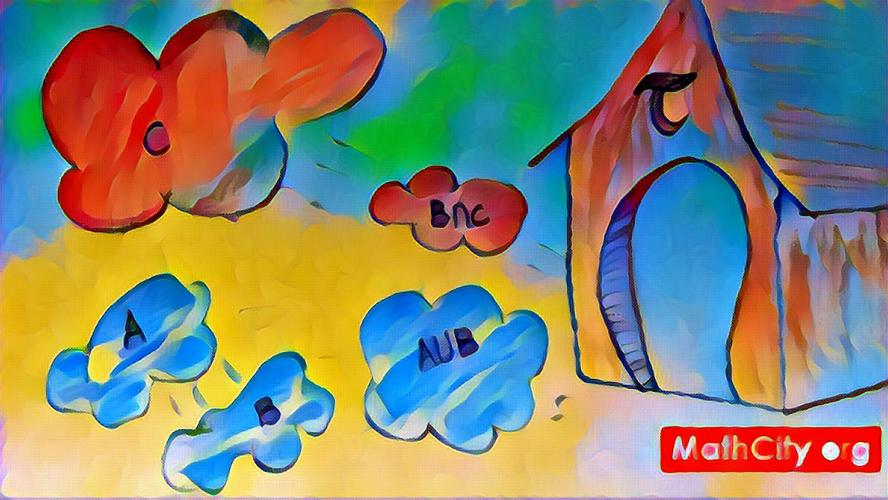# MTH251: Set TopologyTopology is an important branch of mathematics that studies all the “qualitative” or “discrete” properties of continuous objects such as manifolds, i.e. all the properties that aren't changed by any continuous transformations except for the singular (infinitely extreme) ones.

In this sense, topology is a vital arbiter in the “discrete vs continuous” wars. The very existence of topology as a discipline shows that “discrete properties” always exist even if you only work with continuous objects. On the other hand, topology always assumes that these features are “derived” – they're some of the properties of objects and these objects are deeper and that may have many other, continuous properties, too. The topological, discrete properties of these objects are just projections or caricatures of the “whole truth”. (continue reading ...)

At the end of this course the students will be able to understand the theory of metric spaces and topological spaces. They are expected to learn how to write, in logical manner, proofs using important theorems and properties of metric spaces and topological spaces. Students learn to solve problems using the concepts of topology. They present their solutions as rigorous proofs written in correct mathematical English. Students will be able to devise, organize and present brief solutions based on definitions and theorems of topology. Students are expected not only to grasp the concepts of topology and apply them, but also to continue with their overall mathematical development. They will be improving such skills as mathematical writing and the presentation of rigorous logical arguments.

Preliminaries, Metric spaces: Open and closed sets, convergence, completeness.

Continuous and uniformly continuous mappings. Pseudometrics. Fixed point theorem for metric spaces; Topological Spaces. Open bases and sub-bases. Relative topology, Neighborhood system, Limit points, First and second countable spaces. Separable spaces. Products of spaces, Interior, Exterior, Closure and Frontier in product spaces.

Open and closed maps, Continuity and Homeomorphisms, Quotient spaces; Housdorff spaces, regular, and normal spaces, Urysohn's Lemma; Compact spaces, Tychonoff's theorem and locall compact spaces, Compactness for Metric spaces; Connected spaces, Components of a space, Totally disconnected spaces, Local connectedness, Path-wise connectedness

Topological spaces: Definitions

• Define topology on a set.
• Define open set.
• What is discrete topological space?
• What is usual topology on $\mathbb{R}$?
• What is indiscrete topology?
• What is cofinite topology or $T_1$-topology?
• Define closed set.
• Write three open sets and three closed set of the cofinite topology on $\mathbb{Z}$.
• Prove that intersection of topological spaces is topological space.
• Give an example of topological spaces such that their union is not topological space.
• Define accumulation points or define limit-point.
• Define derive set.
• Find the derive set of $A=\{1,2,3,...,20\}$ under the usual topology on $\mathbb{R}$.
• What is the derive set of $\mathbb{Q}$ under the usual topology on $\mathbb{R}$?
• Consider the set $A=\left\{1,\frac{1}{2},\frac{1}{3},... \right\}$. Find the derive set of $A$ under usual topology.
• Define closure of a set.
• Define dense set.
• Define interior point, exterior point and boundary point.
• Under the usual topology on $\mathbb{R}$, find interior and closure of the following sets:
• (i) $A=\mathbb{N}$ (ii) $B=\{1,2,3,...,100\}$ (iii) $C=[-1,1]$
• (iii) $D=(0,5]$ (iv) $E=\{1,2,3\}\cup[4,5]$ (vi) $F=(3,10)$ (vii) $G=\mathbb{Q}$
• Under the discrete topology on $\mathbb{R}$, write interior and closure of the following sets:
• (i) $A=\{1,2,...,10\}$ (ii) $B=[0,1)$ (iii) $C=\mathbb{Q}$
• Define relative topology.
• Let $X=\{a,b,c,d\}$ and $\tau=\{\varphi, X, \{a\}, \{a,c\}\}$. Then find the relative topology of $A=\{c,d\}$.
• Let $A$ be a subset of topological space $X$. Then prove that $A$ is closed in $X$ iff $A'\subset A$.
• Let $A$ be a subset of topological space. Then prove that $A\cup A'$ is closed.
• Let $A$ be a subset of topological space. Then prove that $\overline{A}=A\cup A'$
• Let $A$ and $B$ be subsets of topological space. Then prove that $\overline{A\cup B}=\overline{A}\cup \overline{B}$.

List of Problems taken from :

Page 73-82: Problems 1, 3, 7, 10, 11, 12, 13, 14, 15, 17, 18, 19, 21, 23, 25, 26, 27, 28, 30, 34, 43
1. James Munkres, Topology (2nd Edition), Prentice Hall, 2000.
1. Sheldon Davis, Topology, McGraw-Hill Science/Engineering/Math, 2004.
2. Seymour Lipschutz, Schaum's Outline of General Topology, McGraw-Hill, 2011.
3. G.F. Simmons, Introduction to Topology and Modern Analysis, Tata McGraw-Hill, 2004. (link)
4. Stephen Willard, General Topology, Dover Publications, 2004. (link)
5. M.A. Armstrong, Basic Topology, Springer, 2010.
• atiq/sp18-mth251